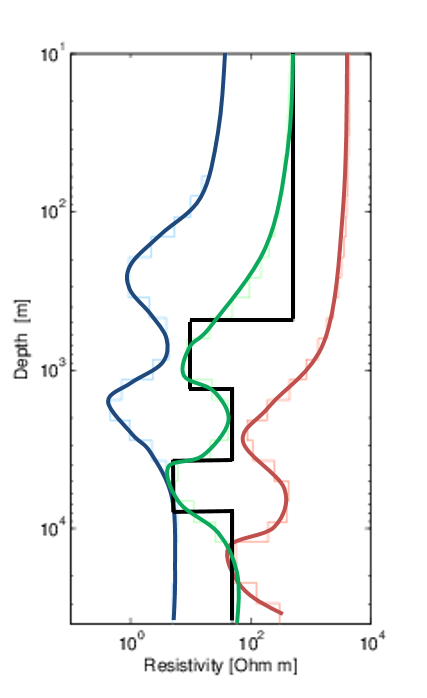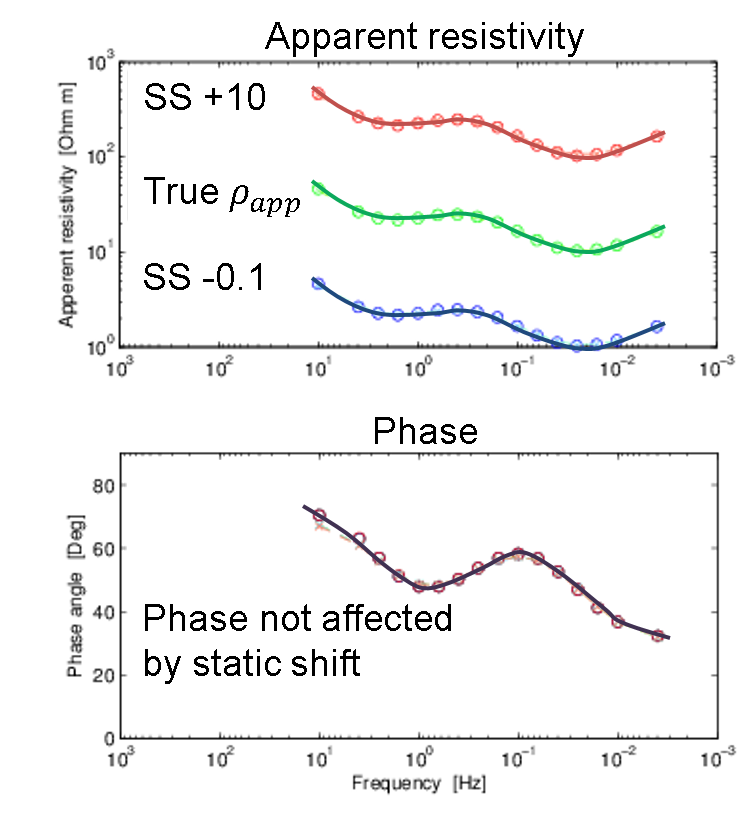Practical Considerations

This page is under construction. Have suggestions or want to contribute? Get in touch!

The static shift is a DC shift in the MT data which is caused by near-surface resistivities close to the receivers, current channeling, and/or topography. It only affects the electric field but causes the apparent resistivity to be shifted (by a multiplier), and can thus provide a false interpretation. It does not affect the phase calculated from the impedance tensor.

Consider the synthetic 1D example in Fig. 207. The true resistivity model is shown in black. The apparent resistivity (in green) and phase (in purple) for this model are shown in Fig. 208. Now consider a static shift that multiplies the apparent resistivity by 10 (shown in red in Fig. 207). The phase is not affected. If these data are inverted, the inversion recovers a model (red in Fig. 207) that does not represent the true model.

Similarly, if the static shift reduced the apparent resistivity by 0.1 (shown in blue in Fig. 208), then the inversion recovers a model that is too conductive compared to the true model (blue in Fig. 207).

Thus, in order to recover a decent model, such as the green model (Fig. 207), MT data may need to be corrected for static shift.Fig. 207 Static shift can cause the inversion to recover a model incorrectly, as shown here. The true model (black) is compared to the recovered models when there is no static shift (green), when the static shift multiplier is 10 (red), and when the static shift multiplier is 0.1 (blue).Fig. 208 The static shift affects only the apparent resistivity and not the phase. The top plot shows how the apparent resistivity changes for static shift multipliers of 10 (red) and 0.1 (blue) compared to the actual data (green).

There are various sources in the literature that discuss removal of the static shift from MT data [SB05], [Ros11] including using additional time-domain EM data to jointly recover the resistivity structures at depth.